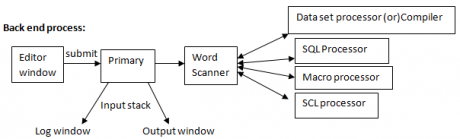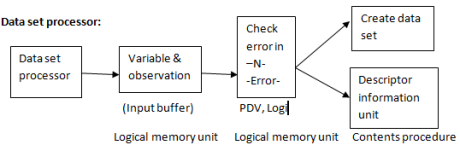# Conditional operators in SAS

Blog Author

Tekslate

Published Date

21st September, 2018

Ratings

Views

1089

### Conditional operators in SAS

1. Conditional (or) comparison operators:

Conditional operators in SAS

 Symbol Mnemonics > gt < lt >= Grater than equal to <= Less than equal to = Equal to ~= Negation equal to

x

### 2.Logical operator:

 Symbol Mnemonics & And | Or (between in not in like)

### Different conditional statements:

1. Where statement(expression)
2. If –else statement
3. If –else if – else if ….else statement
4. Do block
5. Do while loop
6. Do until loop
7. Do loop

### Where statement(expression):

Using where statement, we can create subset of data for reporting (temporary) and storage(permanent). If we write where statement in procedure block its create subset data for reporting and data set block for storage. Ex: Data company; Input cname \$  Area \$ invest strength; Cards; Satyam   Vizag        2000000   567 TCS          HYD          5000000   956 CTS          HYD           6000000   345 TCS          Madras     5000000   678 Wipro        HYD         7000000   789 Wipro      Bngl           6700000   456 Satyam    Bngl           6700000   453 Wipro       Pune         6700000   789 HSBC       HYD            8900000    673 ICICI         Bngl            8000000   898 HDFC       Pune            9000000   796 ; /* Reporting*/ Ex: Proc print data = company; Where cname = ‘Satyam’; Run; /* Storage*/ Ex; Data Satyam; Set company; Where Cname =’Satyam’; Run;

`Accelerate Your Career With SAS Training and become expertise SAS Developer`

### Logical error:

If condition or logical is wrong in statement, then your conditional required data not available in data set. Then in this case we will get logical error. This is one type of execution error. Indication: No observation are selected it is the indication of logical error. Ex: /* Reporting */ Proc print data = company; Where Cname =’Satyam’ and invest ge 6000000; Run; Ex: /* Reporting */ Proc print data = company; Where Cname =’Satyam’ or Cname =’TCS’ Run; Ex: /* Reporting */ Proc print data = company; Where invest between 6000000 and 8000000 Run; Ex: /* To report all company data except Satyam */ Proc print data = company; Where Cname   ne ’Satyam’ ; Run; Ex: /* To report all company data except Satyam and TCS*/ Proc print data = company; Where Cname   ne ’Satyam’ or Cname  ne ‘TCS’ ; Run; Ex: Proc print data =company; Where Cname  ne ’Satyam’ and  Cname  ne  ‘TCS’; Run;   Note: If we use ‘or’ instead of ‘and’ it shows overall data in output. Ex: /* to report Satyam or TCS */ Proc print data = company; Where (Cname eq ’Satyam’ or Cname eq ‘TCS’) And invest >= 5000000; Run; (or) Where invest >= 5000000 and (Cname eq ’Satyam’ or Cname eq ‘TCS’); Ex: Proc print data = company; Where invest between 2000000 and 5000000 ; Run; (or) Proc print data = company; Where invest >=2000000 and invest<=5000000 ; Run; (or) Proc print data = company; Where 2000000 <= invest <=5000000 ; Run;   Ex: Proc print data = company; Where invest  >= 5000000 ; (or) Where 5000000 <= invest; Run; Ex: Proc print data = company; Where (Cname ne ’Satyam’ and Cname ne ‘TCS’) And invest ge 5000000; Run; /* To report numeric missing value */ Ex; Proc print data =company ; Where invest eq; Run; /* To report non – missing numeric value */ Proc print data =company; Where invest  ne; Run; /*To report character memory */ Ex: Proc print data =company; Where Cname eq  ‘   ‘  or area  eq  ‘    ‘; Run; Ex: Proc print data =company; Where Cname = ‘   ‘  ; Run; /* To report non –missing observations based on all variables in data set */ Ex; Proc print data =company; Where Cname ne ‘   ‘ And  area  ne  ‘  ‘ And    invest   ne   . And   strength  ne  .  ; Run; /* To report missing observation  */ Ex: Proc print data =company; Where Cname eq  ‘   ‘ Or area  eq  ‘  ‘ or    invest   eq   . or strength  eq .  ; Run;

### Like operator:

Using like operator, we can produce the result based on part of the matching like operator can be used only for character variable. Percentage %  :  indicates  ‘n’ number of characters. Underscore(_) : indicates only one characters   Ex: Proc print data =company; Where area like ‘p----’; Run; Ex: Proc print data =company; Where Cname  eq ‘ HSBC  ‘  ; Run; Ex: Proc print data =company; Where Cname like  ‘%l’; Run;

### In operator:

It can be used of ‘or’ operator for required variable. ‘In’ indicates to required values not in non required value. Ex; Proc print data =company; Where Cname = ‘ TCS  ‘  or  Cname = ‘HSBC’  ; Run; Proc print data =company; Where Cname in (‘TCS’, ‘HSBC’); Run; Ex: Proc print data =company; Where Cname  not  in (‘TCS’, ‘HSBC’); Run;### Input stack:

Whenever we submit application, SAS convert into the binary format and stores in one logical memory unit in SAS. This unit is called input stack.

### Process:

Input stack connected to the word scanner. Word scanner connected to the 4 compiler. Word scanner work is to identify word value and send to required word to required compiler. Compiler do the compilation and resend to the compilation coding to word scan. Word scan resend to the input stack input stack execute the program.### Input Buffer:

When SAS system read the input statement, it creates input buffer (LMU) brings variable names and observation.

### Program data vector(pdv):

Pdv connected to the input buffer and brings at a time only one observation from input buffer and check the data errors in that observation using 2 automatic variables. –N- , - error. -N- : indicates the number of observations (or) iteration -error- : if value is 0 , then there is no error If value is 1, then error is occurred. Default can be seen by 2 variables in log window whenever error is occurred. This is LMU. Pdv error checking is completed in raw data, pdv creates and stores the variables and assign required data values for data variables. This is called data set unit.

### Descriptor information unit:

This unit is created by the pdv SAS system default stores some information about each and every data set. This information is called descriptor information. If we want to see the information we should use contents procedure. proc contents data = demo; Run;

### If else statement:

It can be used to run required statement based on condition. Ex: Data  ‘emp’; Set emp; If sale ge 500 then salary – salary +1000; Else salary = salary+500; Run; Proc print data = emp; Run;

### If else if else if …else  statement:

Conditions are more we can join else if statement in if else block. Ex: Data  ‘emp’; Set emp; If sale ge 500 then salary – salary +2000; Else if  sale ge 400 and Sale lt 500 then Salary = salary +1500; Else if sale ge 300 and Sale lt 400 then Salary = salary +1000; Elase salary = salary +500; Run; Proc print data = emp; Run; Based on if statement , we can run only one statement. If we want to run multiple statement we use do block with if statement. Do block with end statement.

### Do block:

Ex: Data emp1; Set emp; If sale ge then do; Nsalary = salary +2000; Bonus =1000; End; Else if sale ge 400 and Sale lt 400 then do; N salary = salary +1000; Bonus =500; End; Else do; N salary = salary +500; Bonus =300; End; run; ex: data medi; input pid d level; cards; 100  0.9 101   0.3 102   0.2 103  0.5 104   0.7 105  0.4 ; Data medi; Set medi; If dlevel le 0.5 then do; Drug =’col5mg’; Status =’Normal level’; End; Else if 0.5 < d level <= 0.7 Then do; Drug =’col 10 mg’; Status = ‘middle level’; End; Else do; Drug =’col 15mg’; Status = ‘high level’; End; Proc print data = medi; Run;

### Loop concept:

It can be used to run required statement multiple times. It must have 3 requirements.

1. Loop variable and some value
2. Condition
3. Increment

Do while loop:       Loop concept mainly to generate data. Do while loop to run the loop while condition is true or until the condition is false.   Data generation: Using loop concept,We can generate data.   Out put statement: It can be used to store current observation in current data set.   Syntax: Output<data set name>; Ex: Data one; X=10; output; X=5 ; output; Run; Proc print data =one; run;   /* to store required observation in required data sets using with output statement*/ Ex; Data one two; X=10; output; X=5 ; output; Run; Proc print data =one; run; Proc print data =two; run; ex; data dos1 dos 2 dos 3; set medi 1; if drug =’col 5 mg’ then output dos1; else if drug =’col10 mg’ then output dos2; else output dos3; run; Proc print data =dos1; run; For indepth knowledge on SAS, click on below